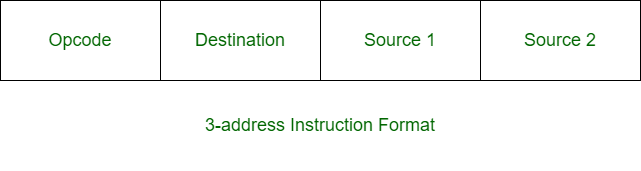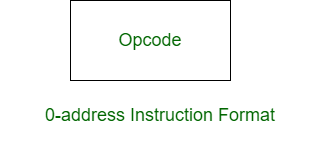Open in App
Not now

• Difficulty Level : Easy
• Last Updated : 16 May, 2020

Prerequisite – Instruction Formats
Three-address instruction is a format of machine instruction. It has one opcode and three address fields. One address field is used for destination and two address fields for source.Example:

`X = (A + B) x (C + D) `

Solution:

```ADD R1, A, B      R1 <- M[A] + M[B]
ADD R2, C, D      R2 <- M[C] + M[D]
MUL X, R1, R2     M[X] <- R1 x R2 ```

Zero-address instruction is a format of machine instruction. It has one opcode and no address fields.Example:

`X = (A + B) x (C + D) `

Solution:

```LOAD A      AC <- M[A]
PUSH A      TOS <- A
PUSH B      TOS <- B
ADD         TOS <- (A + B)
PUSH C      TOS <- C
PUSH D      TOS <- D
ADD         TOS <- (C + D)
MUL         TOS <- (C + D) x (A + B)
POP X       M[X] <- TOS ```